Home > CCA2 > Chapter 12 > Lesson 12.2.2 > Problem12-118

12-118.
1. Prove that each of the following equations is an identity. In other words, prove that they are each true for all values for which the functions are defined. Homework Help ✎

1. (sin θ + cos θ)2 = 1 + 2 sin θ cos θ

2. tan θ + cot θ = sec θ csc θ

3. (tan θ cos θ)(sin2θ +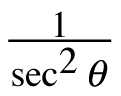) = sin θ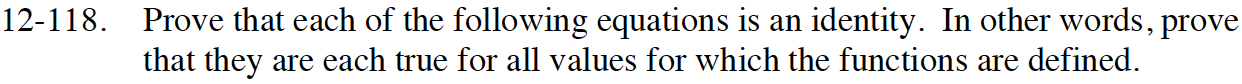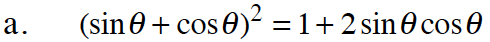Start by expanding the left side of the equation.
You might have to rearrange the equation to see ways to simplify it after you expand it.

Change the trigonometric identities on the left side of the equation into the ratios they represent.

$\frac{\sin\theta}{\cos\theta}+\frac{\cos\theta}{\sin\theta}=$

Find a common denominator and then add the two terms.

$\frac{\sin^{2}\theta}{\cos\theta\sin\theta}+\frac{\cos^{2}\theta}{\cos\theta\sin\theta}=$

Remember that sin²(θ) + cos²(θ) = 1, and rewrite the equation.

$\frac{1}{\cos\theta\sin\theta}=$

Substitute in the identities for the two terms.
What does 1/cosθ equal? 1/sinθ?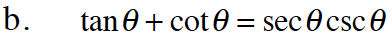Substitute the trig identities in first, then expand by multiplying the two terms together.
Remember to use Giant Ones to simplify when you can.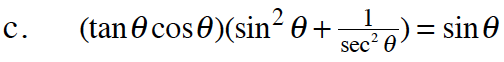Use methods from both parts (a) and (b).